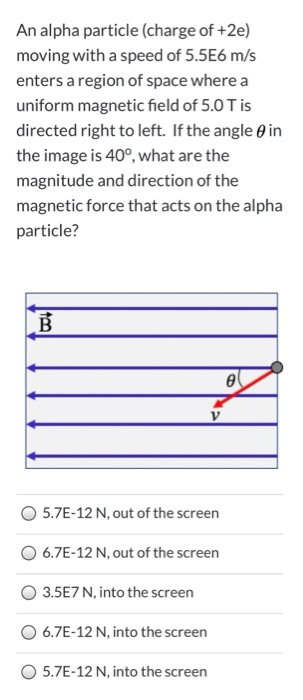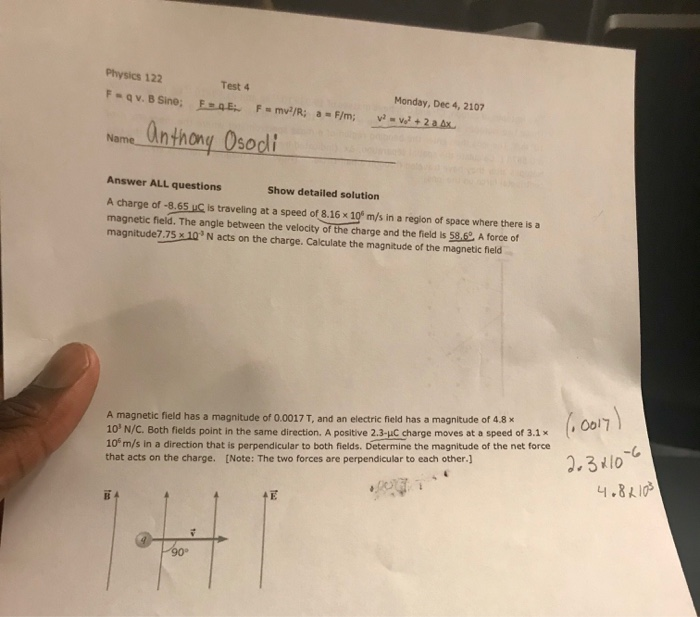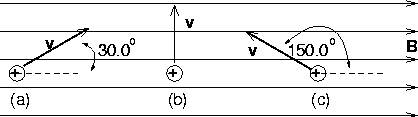# A charge of -6.09 μC is traveling at a speed of 6.65 × 106 m/s in...

A charge of -6.09 μC is traveling at a speed of 6.65 × 106 m/s in a region of space where there is a magnetic field. The angle between the velocity of the charge and the field is 53.4o. A force of magnitude 8.59 × 10-3 N acts on the charge. What is the magnitude of the magnetic field?

F = q x V x B x Sin 53.4o

8.59 x 10-3 = 6.09 x 10-6 x 6.65 x 106 x B x Sin 53.4o

Solving, we get : B = 2.64 x 10-4 T Answer

#### Earn Coin

Coins can be redeemed for fabulous gifts.

Similar Homework Help Questions
• ### A charge of -5.92 μC is traveling at a speed of 5.16 × 106 m/s in...

A charge of -5.92 μC is traveling at a speed of 5.16 × 106 m/s in a region of space where there is a magnetic field. The angle between the velocity of the charge and the field is 57.4o. A force of magnitude6.76 × 10-3 N acts on the charge. What is the magnitude of the magnetic field?

• ### A charge of -8.81 μC is traveling at a speed of 5.03 × 106 m/s in...

A charge of -8.81 μC is traveling at a speed of 5.03 × 106 m/s in a region of space where there is a magnetic field. The angle between the velocity of the charge and the field is 51.1o. A force of magnitude6.47 × 10-3 N acts on the charge. What is the magnitude of the magnetic field?

• ### A charge of -5.16 μC is traveling at a speed of 5.36 × 106 m/s in...

A charge of -5.16 μC is traveling at a speed of 5.36 × 106 m/s in a region of space where there is a magnetic field. The angle between the velocity of the charge and the field is 53.3o. A force of magnitude8.99 × 10-3 N acts on the charge. What is the magnitude of the magnetic field?

• ### A charge of -7.4 µC is traveling at a speed of 8.6 106 m/s in a...

A charge of -7.4 µC is traveling at a speed of 8.6 106 m/s in a region of space where there is a magnetic field. The angle between the velocity of the charge and the field is 52°. A force of magnitude 4.7 10-3 N acts on the charge. What is the magnitude of the magnetic field?

• ### A particle with a charge of 38 μC moves with a speed of 73 m/s in...

A particle with a charge of 38 μC moves with a speed of 73 m/s in the positive x direction. The magnetic field in this region of space has a component of 0.44 T in the positive y direction, and a component of 0.84 T in the positive z direction. What is the magnitude of the magnetic force on the particle? What is the direction of the magnetic force on the particle? (Find the angle measured from the positive z-axis...

• ### A particle with a charge of 36 μC moves with a speed of 71 m/s in...

A particle with a charge of 36 μC moves with a speed of 71 m/s in the positive x direction. The magnetic field in this region of space has a component of 0.39 T in the positive y direction, and a component of 0.84 T in the positive z direction. Part A What is the magnitude of the magnetic force on the particle? Express your answer using two significant figures. Part B What is the direction of the magnetic force...

• ### An alpha particle (charge of +2e) moving with a speed of 5.5E6 m/s enters a region...An alpha particle (charge of +2e) moving with a speed of 5.5E6 m/s enters a region of space where a uniform magnetic field of 5.0 T is directed right to left. If the angle in the image is 40°, what are the magnitude and direction of the magnetic force that acts on the alpha particle? B e V 5.7E-12 N, out of the screen 6.7E-12 N, out of the screen 3.5E7 N, into the screen 6.7E-12 N, into the screen...

• ### Physics 122 Test 4 Monday, Dec 4, 2107 Name Anthong Osod Answer ALL questions Show detailed...Physics 122 Test 4 Monday, Dec 4, 2107 Name Anthong Osod Answer ALL questions Show detailed solution A charge of -8.65 uC Is traveling at a speed of 8.16x 10° m/s in a region of space where there is a magnetic field. The angle between the velocity of the charge and the field is 58.6 A forcef magnitude7.75 x 10 N acts on the charge. Calculate the magnitude of the magnetic field A magnetic field has a magnitude of 0.0017...

• ### (a) A proton, traveling with a velocity of 3.5 106 m/s due east, experiences a magnetic...

(a) A proton, traveling with a velocity of 3.5 106 m/s due east, experiences a magnetic force that has a maximum magnitude of 7.1 10-14 N and a direction of due south. What are the magnitude and direction of the magnetic field causing the force? magnitude     T (b) Repeat part (a) assuming the proton is replaced by an electron. magnitude T

• ### Three particles, each with a charge of 4.75 μC and a speed of 365.0 m/s, enter...Three particles, each with a charge of 4.75 μC and a speed of 365.0 m/s, enter a uniform magnetic field whose magnitude is 0.325 T. What is the magnitude of the magnetic force on particle a? What is the magnitude of the magnetic force on particle b? What is the magnitude of the magnetic force on particle c? 30.0 v 150.0